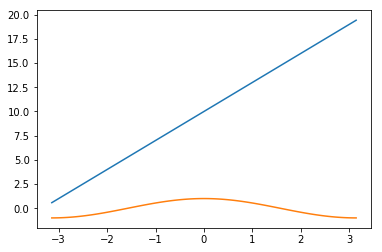In :
import numpy as np


# Creating arrays in NumPy¶

In :
#create an array, betwen 1 and 2, with a step of 0.01
r = np.arange(1,2, 0.01)
print(r)
r.sum()

[1.   1.01 1.02 1.03 1.04 1.05 1.06 1.07 1.08 1.09 1.1  1.11 1.12 1.13
1.14 1.15 1.16 1.17 1.18 1.19 1.2  1.21 1.22 1.23 1.24 1.25 1.26 1.27
1.28 1.29 1.3  1.31 1.32 1.33 1.34 1.35 1.36 1.37 1.38 1.39 1.4  1.41
1.42 1.43 1.44 1.45 1.46 1.47 1.48 1.49 1.5  1.51 1.52 1.53 1.54 1.55
1.56 1.57 1.58 1.59 1.6  1.61 1.62 1.63 1.64 1.65 1.66 1.67 1.68 1.69
1.7  1.71 1.72 1.73 1.74 1.75 1.76 1.77 1.78 1.79 1.8  1.81 1.82 1.83
1.84 1.85 1.86 1.87 1.88 1.89 1.9  1.91 1.92 1.93 1.94 1.95 1.96 1.97
1.98 1.99]

Out:
149.50000000000003
In :
#array has a shape. (3) = one column (Vector) ; (3,2) = two columns (Matrix); (3,2,2) = tensor

#Create three vectors for 3 users; each of which rate three films
me = np.array([8, 5, 9])
you = np.array([3, 9, 6])
bob = np.array([1, 7, 4])

print(me)
print(you)
print(bob)

[8 5 9]
[3 9 6]
[1 7 4]


# Create a matrix from multiple arrays¶

In :
#create a matrix
users = np.array([me, you, bob])
print(users)

[[8 5 9]
[3 9 6]
[1 7 4]]


# Calculate dot product between two vectors (how similar are they)¶

In :
#calculate the dot product
#how similar are two users. How similar is me to you and me to bob?

me = np.array([8, 5, 9])  #each matrix needs to be normalised to get actual angle between the two vectors
you = np.array([3, 9, 6])
bob = np.array([1, 7, 4])

print(me.dot(you))
print(me.dot(bob))

123
79


# Use linspace as an alternative method of generating data¶

In :
#similar to np.arange(-10, 10, 1), except we define how many points we want, rather than the step size
np.linspace(-10, 10, 10)

Out:
array([-10.        ,  -7.77777778,  -5.55555556,  -3.33333333,
-1.11111111,   1.11111111,   3.33333333,   5.55555556,
7.77777778,  10.        ])
In :
import seaborn as sns

#generate 100 plots between -3.14 and +3.14
x = np.linspace(-np.pi, np.pi, 100)
y = np.cos(x)

def myfunction(x):
return 3*x+10

sns.lineplot(x, myfunction(x))
sns.lineplot(x, y)

Out:
<matplotlib.axes._subplots.AxesSubplot at 0x1a1e7713c8>In :
x = np.array([[2,0],
[0,2]])


# functions:¶

In :
def y(x):
a = 5
b = 3
c = 1

return a * x[:,0] + b * x[:,1] + c

In :
y(x)

Out:
array([11,  7])

# Join multiple vectors to be a matrix & then do slicing¶

In :
user1 = np.array([2,0,3])
user2 = np.array([6,1,7])
user3 = np.array([4,3,2])
matrix = np.array([user1,user2,user3])

In :
matrix

Out:
array([[2, 0, 3],
[6, 1, 7],
[4, 3, 2]])
In :
#print first column of matrix
matrix[:,0]

Out:
array([2, 6, 4])
In :
#print last two columns for matrix
matrix[:,-2:]

Out:
array([[0, 3],
[1, 7],
[3, 2]])
In :
#print first two users for matrix
matrix[:2,]

Out:
array([[2, 0, 3],
[6, 1, 7]])
In :
#return only values that correspond to true
user2[[ True, True, False]]

#return elements greater than 3
user2[ user2 > 3]
user2[ user2 > 3]
user2[ user2 == 7]

Out:
array()
In :
#what films that user 1 rated at > 1 - what did user 2 rate them at
user2[ user1 > 1]

Out:
array([6, 7])

# Pandas Intro¶

In :
import pandas as pd
df = pd.DataFrame({
"HR": np.linspace(60,120,10),
"BP": np.linspace(90, 130, 10),
"y": [-1, 1] * 5
})

In :
df

Out:
HR BP y
0 60.000000 90.000000 -1
1 66.666667 94.444444 1
2 73.333333 98.888889 -1
3 80.000000 103.333333 1
4 86.666667 107.777778 -1
5 93.333333 112.222222 1
6 100.000000 116.666667 -1
7 106.666667 121.111111 1
8 113.333333 125.555556 -1
9 120.000000 130.000000 1
In :
#second row HR
df.loc[2, 'HR']
#all rows in HR
df.loc[:, 'HR']

Out:
0     60.000000
1     66.666667
2     73.333333
3     80.000000
4     86.666667
5     93.333333
6    100.000000
7    106.666667
8    113.333333
9    120.000000
Name: HR, dtype: float64
In :
#extract out as np array
df['HR'].values

Out:
array([ 60.        ,  66.66666667,  73.33333333,  80.        ,
86.66666667,  93.33333333, 100.        , 106.66666667,
113.33333333, 120.        ])
In [ ]: